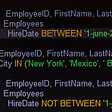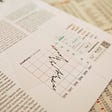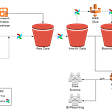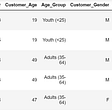# Transform data

• last name (nom)
• first names (prenom)
• gender (sexe)
• birth date (date_naissance)
• City of the birth (for people born in France or in DOM/TOM/COM) (ville_naissance)
• Country of the birth (for people born abroad) (pays_naissance)
• Death date (date_deces)
• Code of the death location (code_lieu_deces)
• Number of the death certificate (num_acte_deces)
`import pandas as pddf = pd.DataFrame()for a in range(1970, 2022):    dfnew = pd.read_excel('<YOUR DIRECTORY>\\deces-' + str(a) + '.xlsx', engine='openpyxl', header=None)    df = df.append(dfnew,ignore_index=True)`
`df.columns = ['name', 'naissance', 'mort']df[['nom', 'prenom']] = df.name.str.split('*' ,expand=True,)df.prenom = df.prenom.str.rstrip('/')df = df.drop(['name'], axis=1)# Birth datadf['sexe'] = df.naissance.strdf['date_naissance'] = df.naissance.str[1:9]df['ville_naissance'] = df.naissance.str[14:44]df['pays_naissance'] = df.naissance.str[44:]df = df.drop(['naissance'], axis=1)# Death datadf['date_deces'] = df.mort.str[0:8]df['code_lieu_deces'] = df.mort.str[9:14]df['num_acte_deces'] = df.mort.str[15:]df = df.drop(['mort'], axis=1)df = df.dropna(subset=['date_naissance', 'date_deces'])`
`df['mois'] = df.date_naissance.str[4:5]df['jour'] = df.date_naissance.str[6:7]df = df[~df.mois.str.contains('00', na=False)]df = df[~df.jour.str.contains('00', na=False)]df = df.drop(['mois', 'jour'], axis=1)df['mois'] = df.date_deces.str[4:5]df['jour'] = df.date_deces.str[6:7]df = df[~df.mois.str.contains('00', na=False)]df = df[~df.jour.str.contains('00', na=False)]df = df.drop(['mois', 'jour'], axis=1)df['date_naissance'] = pd.to_datetime(df.date_naissance, format="%Y%m%d", errors='coerce')df['date_deces'] = pd.to_datetime(df.date_deces, format="%Y%m%d", errors='coerce')df = df.dropna(subset=['date_naissance', 'date_deces'])`
`df['annee'] = df['date_deces'].dt.yeardf['age'] = df.date_deces - df.date_naissancedf['age'] = (df.age.dt.days / 365.25).astype('int')`

# Save our dataframe

`df.to_pickle('<YOURDIRECTORY>\\df.pickle')`

# Oldest persons since 1970

`df = df.sort_values(by='age', ascending=False).groupby(by='annee').first()import matplotlib.pyplot as pltfigure(figsize=(20, 20), dpi=100)df = df.reset_index()df = df[df.annee > 1970]plt.plot(df.annee, df.age)plt.show()`

# Number of death by year

`# We reload the dataframedf = pd.read_pickle('<YOUR DIRECTORY>\\df.pickle')df['date_deces'] = df.date_deces.dt.strftime('%Y')df = df.groupby(by=['annee', 'date_deces']).size().to_frame()df = df.sort_values(by=, ascending=False)df = df.reset_index()# We are drawing the resultimport matplotlib.pyplot as pltfrom matplotlib.pyplot import figurefigure(figsize=(20, 20), dpi=100)df = df[df.annee>1972]df = df.sort_values(by=['annee'], ascending=False)plt.plot(df.annee, df, label='Death by year')plt.show()`

# Population born in an other country

`# We reload the dataframedf = pd.read_pickle('<YOUR DIRECTORY>\\df.pickle')# We use seaborn to draw the barplotimport seaborn as snsfigure(figsize=(20, 20), dpi=100)# We remove the blank country which represent Francedf = df[df['pays_naissance'] != '']group = df.groupby(['pays_naissance']).size()df = group.to_frame(name = 'nb_pays').reset_index()df = df.sort_values(by=['nb_pays'], ascending=False)# We are going to print the 15 firsts resultsdf = df.head(15)sns.barplot(data=df, x=df.pays_naissance, y=df['nb_pays'], label="nb", color="blue")`

# Death age by country of birth

`# We reload the dataframedf = pd.read_pickle('<YOUR DIRECTORY>\\df.pickle')import matplotlib.pyplot as plt# First the overall averagefigure(figsize=(20, 20), dpi=100)group = df.groupby(by='annee')['age'].mean()group = group[group.index > 1992]plt.plot(group.index, group, label='Moyenne globale')plt.legend()# For ALGERIAgroup = df.groupby(by=['annee', 'pays_naissance'])['age'].mean().to_frame()group = group.reset_index()group = group[group['pays_naissance'] == 'ALGERIE']group = group[group['annee'] > 1992]plt.plot(group.annee, group.age, label='ALGERIE')plt.legend()# For MOROCCOgroup = df.groupby(by=['annee', 'pays_naissance'])['age'].mean().to_frame()group = group.reset_index()group = group[group['pays_naissance'] == 'MAROC']group = group[group['annee'] > 1992]plt.plot(group.annee, group.age, label='MAROC')plt.legend().......... Same thing for each country then :plt.plot(group.annee, group.age)plt.show()`

# Death age by month of birth

`# We reload the dataframedf = pd.read_pickle('<YOUR DIRECTORY>\\df.pickle')# We only keep the birth monthdf['date_naissance'] = df.date_naissance.dt.strftime('%m')tab_annee = [j for j in range(1972, 2022)]tab_mois = [j for j in range(1, 13)]tab_moy = []for j in range(1972, 2022):    tab = []    for i in range(1, 13):        if i<10:            mois = '0' + str(i)        else:            mois = str(i)        tab.append(df[(df.date_naissance == mois) & (df.annee == j)].age.mean())    tab_moy.append(tab)dfmoy = pd.DataFrame(tab_moy, columns=tab_mois, index=tab_annee)`
`import matplotlib.pyplot as pltfrom matplotlib.pyplot import figurefigure(figsize=(20, 20), dpi=100)plt.plot(dfmoy.index, dfmoy, label='Janvier')plt.plot(dfmoy.index, dfmoy, label='Février')plt.plot(dfmoy.index, dfmoy, label='Mars')plt.plot(dfmoy.index, dfmoy, label='Avril')plt.plot(dfmoy.index, dfmoy, label='Mai')plt.plot(dfmoy.index, dfmoy, label='Juin')plt.plot(dfmoy.index, dfmoy, label='Juillet')plt.plot(dfmoy.index, dfmoy, label='Aout')plt.plot(dfmoy.index, dfmoy, label='Septembre')plt.plot(dfmoy.index, dfmoy, label='Octobre')plt.plot(dfmoy.index, dfmoy, label='Novembre')plt.plot(dfmoy.index, dfmoy, label='Décembre')plt.legend()plt.show()`

# Month with the more deaths

`# We reload the dataframedf = pd.read_pickle('<YOUR DIRECTORY>\\df.pickle')df['date_deces'] = df.date_deces.dt.strftime('%m')tab_annee = [j for j in range(1972, 2022)]tab_mois = [j for j in range(1, 13)]tab_sum = []for j in range(1972, 2022):    tab = []    for i in range(1, 13):        if i<10:            mois = '0' + str(i)        else:            mois = str(i)        tab.append(len(df[(df.date_deces == mois) & (df.annee == j)].index))    tab_sum.append(tab)dfsum = pd.DataFrame(tab_sum, columns=tab_mois, index=tab_annee)# We are creating the graphimport matplotlib.pyplot as pltfrom matplotlib.pyplot import figurefigure(figsize=(20, 20), dpi=100)plt.plot(dfsum.index, dfmoy, label='Janvier')plt.plot(dfsum.index, dfmoy, label='Février')plt.plot(dfsum.index, dfmoy, label='Mars')plt.plot(dfsum.index, dfmoy, label='Avril')plt.plot(dfsum.index, dfmoy, label='Mai')plt.plot(dfsum.index, dfmoy, label='Juin')plt.plot(dfsum.index, dfmoy, label='Juillet')plt.plot(dfsum.index, dfmoy, label='Aout')plt.plot(dfsum.index, dfmoy, label='Septembre')plt.plot(dfsum.index, dfmoy, label='Octobre')plt.plot(dfsum.index, dfmoy, label='Novembre')plt.plot(dfsum.index, dfmoy, label='Décembre')plt.legend()plt.show()`

# Conclusion

--

--

--

## More from cloud

Love podcasts or audiobooks? Learn on the go with our new app.

## Hands-on experience with BigQuery: how to query with Google Analytics Data## Data Analysis Case Study: Part 1## Principled Data Engineering, Part I: Architectural Overview## Datashare and Workbench: Experimenting Data journalism apps## Predicting customer behavior with Starbucks data## TF-IDF in a nutshell## 8 factors shaping the future of big data, machine learning and AI## Why We Need Higher Sampled Sound## Working with duplicated data in Pandas DataFrame## Getting NSE data using python.## Pandas Tutorial (Part 3)## Data manipulation with Pandas